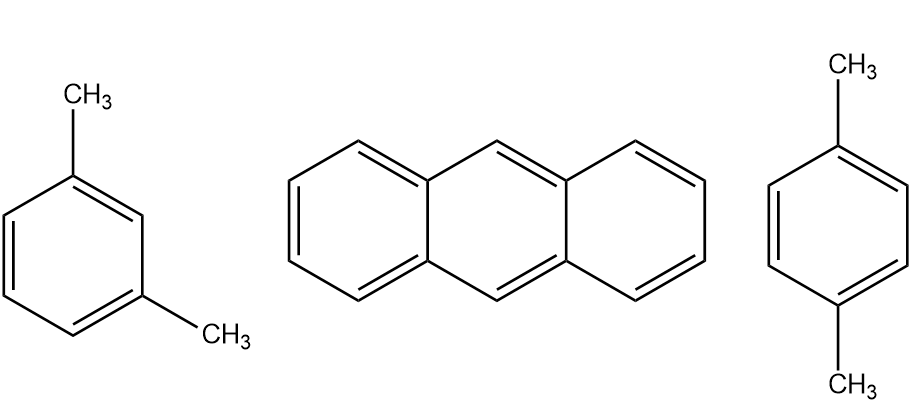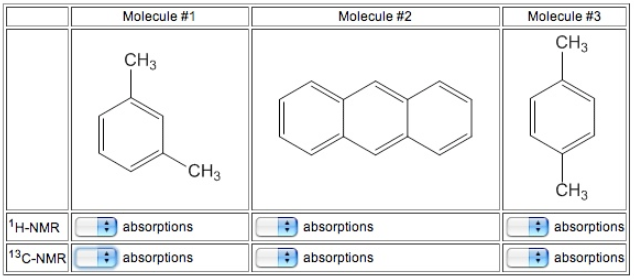# Problem: How many signals would you expect each of the following molecules to have in its 1H and 13C spectra?

###### FREE Expert Solution

For this problem, we need to determine the 1H and 13C signals for each molecule:• Recall that for 1H-NMR analysis there are as many signals on each spectrum as there are unique, non-equivalent protons
• In this case, let's assume that Hs bound to the same C is equivalent
• Take note that symmetry will reduce the total number of signals
88% (414 ratings)###### Problem Details

How many signals would you expect each of the following molecules to have in its 1H and 13C spectra?